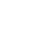# Handling Multiple Streams with Merge

You often need to handle multiple user interactions set to different streams. This lesson shows hows `Observable.merge` behaves like a "logical OR" to have your stream handle one interaction OR another.Vasyl
~ 4 years ago

Hi, I`m trying to start the same code in *.ts file, but receive an error: Error:(43, 18) TS2345:Argument of type '{ count: number; }' is not assignable to parameter of type '((acc: any) => { count: any; }) | ((acc: any) => { count: number; }) | IScheduler'. Type '{ count: number; }' is not assignable to type 'IScheduler'. Property 'now' is missing in type '{ count: number; }'. It is in the string with data param:

startButtonClick\$ .switchMapTo(incOrReset\$) .startWith(data) .scan((acc, curr) => curr(acc)) .subscribe((x)=> console.log(x)); However, this code works in *.js file. Can somebody help me with, how to solve current issue?ganqqwerty
~ 3 years ago

mind-blowing, it really requires a new way of thinking, damn. I'm not sure I understand the diff between mapTo and switchMapTo. Is it correct that mapTo maps the stream to a function whereas switchMapTo maps the stream to another stream?Bogdan
~ 2 years ago

Hi, I`m trying to start the same code in *.ts file, but receive an error: Error:(43, 18) TS2345:Argument of type '{ count: number; }' is not assignable to parameter of type '((acc: any) => { count: any; }) | ((acc: any) => { count: number; }) | IScheduler'. Type '{ count: number; }' is not assignable to type 'IScheduler'. Property 'now' is missing in type '{ count: number; }'. It is in the string with data param:

startButtonClick\$ .switchMapTo(incOrReset\$) .startWith(data) .scan((acc, curr) => curr(acc)) .subscribe((x)=> console.log(x)); However, this code works in *.js file. Can somebody help me with, how to solve current issue?

Hello! Not sure best or not, but i find way to resolve this (I have RxJS version 6.3.3). startStream.pipe( switchMapTo(merge( _interval.pipe(mapTo(inc)), resetStream.pipe(mapTo(data)) )), startWith(data), scan((acc: number, curr:(acc:number)=>number) => curr(acc)) ).subscribe((arg) => { console.log(arg) });Bogdan
~ 2 years ago

UDP: Tips above correct if you use data as number, if data is object, as in lesson above, correct be:

``````startStream.pipe(
switchMapTo(merge(
_interval.pipe(mapTo(inc)),
resetStream.pipe(mapTo(() => 0))
)),
startWith(0),
scan((acc: {count: number}, curr:(acc:{count: number})=>{count: number}) => curr(acc))
).subscribe((arg) => {
console.log(arg)
});``````